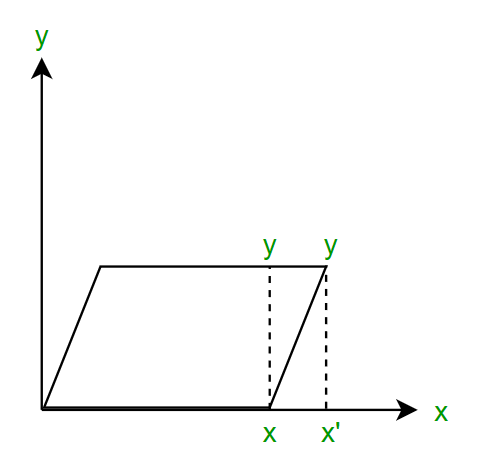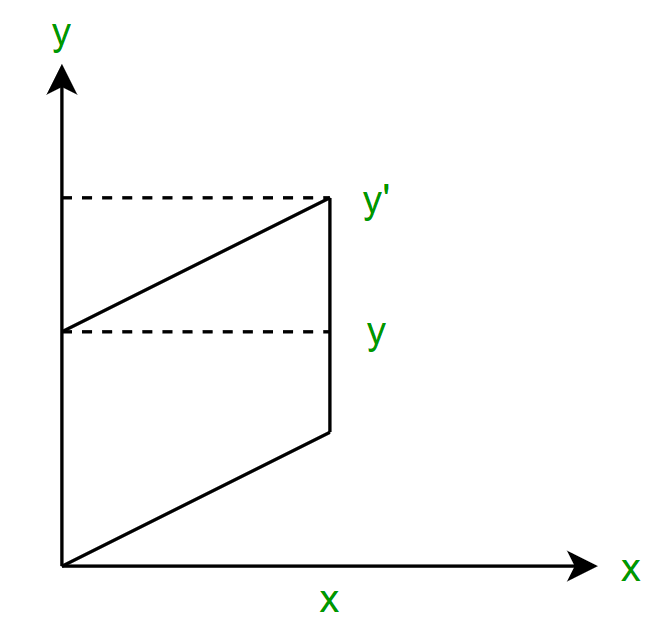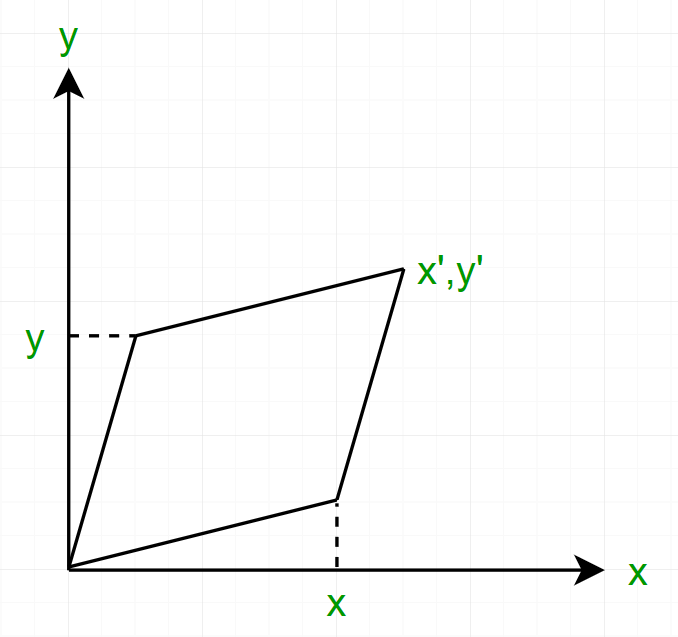Related Articles
Shearing in 2D Graphics
• Difficulty Level : Medium
• Last Updated : 01 Jul, 2020

Shearing deals with changing the shape and size of the 2D object along x-axis and y-axis. It is similar to sliding the layers in one direction to change the shape of the 2D object.It is an ideal technique to change the shape of an existing object in a two dimensional plane. In a two dimensional plane, the object size can be changed along X direction as well as Y direction.

x-Shear :
In x shear, the y co-ordinates remain the same but the x co-ordinates changes. If P(x, y) is the point then the new points will be P’(x’, y’) given as –Matrix Form:y-Shear :
In y shear, the x co-ordinates remain the same but the y co-ordinates changes. If P(x, y) is the point then the new points will be P’(x’, y’) given as –Matrix Form:x-y Shear :
In x-y shear, both the x and y co-ordinates changes. If P(x, y) is the point then the new points will be P’(x’, y’) given as –Matrix Form:Example :
Given a triangle with points (1, 1), (0, 0) and (1, 0).
Find out the new coordinates of the object along x-axis, y-axis, xy-axis.
(Applying shear parameter 4 on X-axis and 1 on Y-axis.).

Explanation –

Given,
Old corner coordinates of the triangle = A (1, 1), B(0, 0), C(1, 0)
Shearing parameter along X-axis (Shx) = 4
Shearing parameter along Y-axis (Shy) = 1

Along x-axis:
A'=(1+4*1, 1)=(5, 1)
B'=(0+4*0, 0)=(0, 0)
C'=(1+4*0, 0)=(1, 0)

Along y-axis:
A''=(1, 1+1*1)=(1, 2)
B''=(0, 0+1*0)=(0, 0)
C''=(1, 0+1*1)=(1, 1)

Along xy-axis:
A'''=(1+4*1, 1+1*1)=(5, 2)
B'''=(0+4*0, 0+1*0)=(0, 0)
C'''=(1+4*0, 0+1*1)=(1, 1) 

Attention reader! Don’t stop learning now. Get hold of all the important CS Theory concepts for SDE interviews with the CS Theory Course at a student-friendly price and become industry ready.

My Personal Notes arrow_drop_up